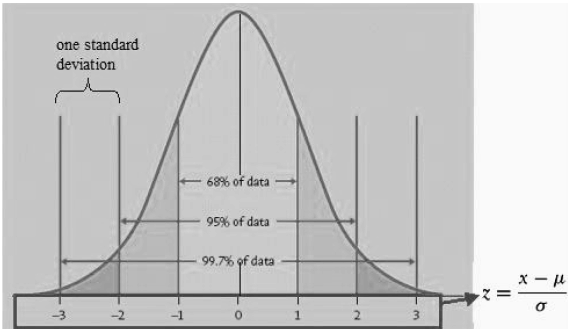# Confidence Intervals for a Normal Distribution

A confidence interval is an interval in which we expect the actual outcome to fall with a given probability (confidence). Consider the following statement:

In a normal distribution, 68% of the values fall within 1 standard deviation of the mean.

So, if X is a normal random variable, the 68% confidence interval for X is -1s <= X <= 1s. This is demonstrated in the following diagram.The four commonly used confidence intervals for a normal distribution are:

• 68% of values fall within 1 standard deviation of the mean (-1s <= X <= 1s)
• 90% of values fall within 1.65 standard deviations of the mean (-1.65s <= X <= 1.65s)
• 95% of values fall within 1.96 standard deviations of the mean (-1.96s <= X <= 1.96s)
• 99% of values fall within 2.58 standard deviations of the mean (-2.58s <= X <= 2.58s)

The confidence interval is generally represented as , where n is the number of standard deviations. For example, n=1.65 for 90% confidence interval.

Example

A stock portfolio has mean returns of 10% per year and the returns have a standard deviation of 20%. The returns are normally distribution. Calculate the 99% confidence interval.

95% confidence interval = 10% +/- 2.58*20%.

The confidence interval is -41.6% to 61.6%. This means with 99% confidence, the returns will range from -41.6% to 61.6%.

Get our Data Science for Finance Bundle for just $29$51.